Courses
Courses for Kids
Free study material
Free LIVE classes
More

# Parabola General Equations - General Equations for JEELIVE
Join Vedantu’s FREE Mastercalss

## An Introduction to Parabola

Parabola is a mathematical curve that is a part of the conic section. It is a plane curve which has a mirror symmetry and is approximately U shaped. The parabola fits several different descriptions in our everyday life and in even superficial situations both in real life and even in Physics. All these situations essentially define the same curve, that is parabola.

The parabola is one of the most important topics under the conic section. A section of a right circular cone cut by a plane which is parallel to the cone generator is basically known as a parabola. Its shape is traced by a quadratic equation and the graph of parabola shows the locus of a point that is always at a fixed distance from a stationary line. A point on the plane also remains fixed.

## What Is Parabola?

The word "parabola" is made up of two words “para” and “bola”. “Para” means "for" and "bola" means "to throw". Therefore, the meaning is self-explanatory. "Parabola" refers to the shape or form of a curve created by kicking or tossing a ball into the air. A parabola can be defined as the locus of a point on a graph which moves in such a way that its distance from a particular fixed point that we call the focus is equal to its perpendicular distance from a fixed straight line called the directrix. The parabola is an important curve in coordinate geometry's conic sections.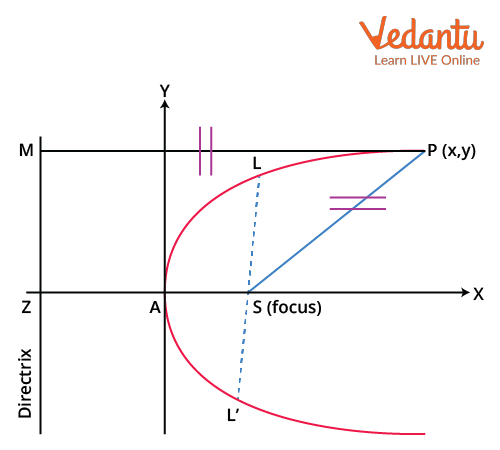Diagram of a Parabola

From the diagram above, we can see that S is the focus and ZM is the directrix. We have considered a point P on the parabola. So from our definition of the parabola we can write

PS=PM

Here PM is the perpendicular distance of the point from the directrix and PS is the distance of the point from the focus.The Parabola in Real Life Scenario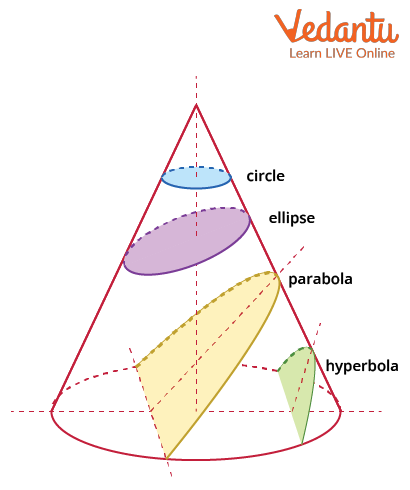Different Curves from a Conic Section

From the image above, we can visualise the parabola as a part of the conic section. Other conic sections include the circle, ellipse, and the hyperbola. All the conic sections have their own properties.

## Important Definitions Related to the Parabola

Some important definitions have been described concerning the parabola, which are as follows:

S.No.

Terminology

Definition

The focus of the Parabola

A parabola is defined by a set of all points in a plane which are at equal distance from a given point and given line. The point is called the focus of the parabola.

Directrix of the Parabola

A parabola is defined by a set of all points in a plane which are at equal distance from a given point and given line. The line is called the directrix of the parabola.

Vertex

The vertex is the point at which the parabola and the axis of symmetry cross each other. The parabola curves dramatically at the vertex.

Focal Length

On the axis of symmetry, it is the distance between the vertex and the focus.

Latus Rectum

It's a line segment that runs parallel to the directrix and passes through the parabola's focus.

Axis of Symmetry

The line of symmetry of a parabola is a line that divides the parabola into two equal sections. It travels through the focus and is perpendicular to the directrix.

Eccentricity

Eccentricity is the ratio of a point's distance from the directrix to its distance from the focus. The parabola's eccentricity is 1.

The terms used in the above table have been depicted in the graph below.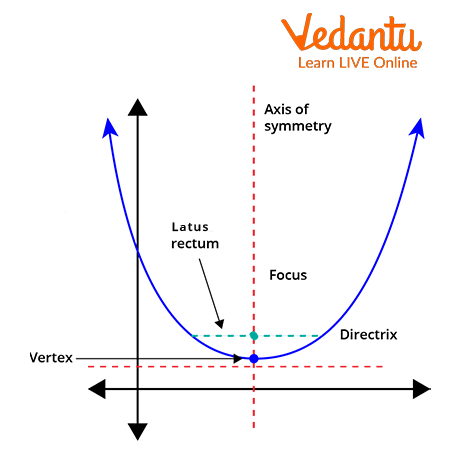Parts of a Parabola

## General Equation of the Parabola

Here are the general equations of the parabola:

$y=a(x-h)^2+k$

$x=a(y-k)^2+h$

From the equations, we can deduce the nature of the parabola. The value of “a” in the equation of the parabola defines the direction of the parabola. The first equation is the equation of a regular parabola that opens towards the y axis. If the value of a is negative then the parabola opens towards the negative y axis and if it is positive then it opens towards the positive y axis.

The second equation is the equation of a sideways parabola that opens towards the x axis. Again, if a is positive then the parabola opens towards the positive x axis and if it is negative then it opens towards the negative x axis.

For a sideways parabola, we have some parabola formulas as listed below that help in deducing the important point of the parabola.

• (h,k) defines the vertex of the parabola.

• Latus rectum of the parabola is equal to 4a.

• The coordinates of the focus are  $\left(h,k+\dfrac{1}{4a}\right)$

• The directrix of the parabola is the line drawn perpendicular to the x-axis and passing the point (-a, 0). The directrix is perpendicular to the parabola's axis.

## Derivation of the Parabola Equation

On the given parabola, there is a point P with the coordinates (x, y). The distance between point P and the directrix is equal to the distance between point P and the focus F, according to the definition of a parabola.

The eccentricity of the parabola is equal to 1. From the definition of parabola mentioned before, we can write the eccentricity e for the parabola as

$\dfrac{PB}{PF}=e$

$\dfrac{PB}{PF}=1$

$PB=PF$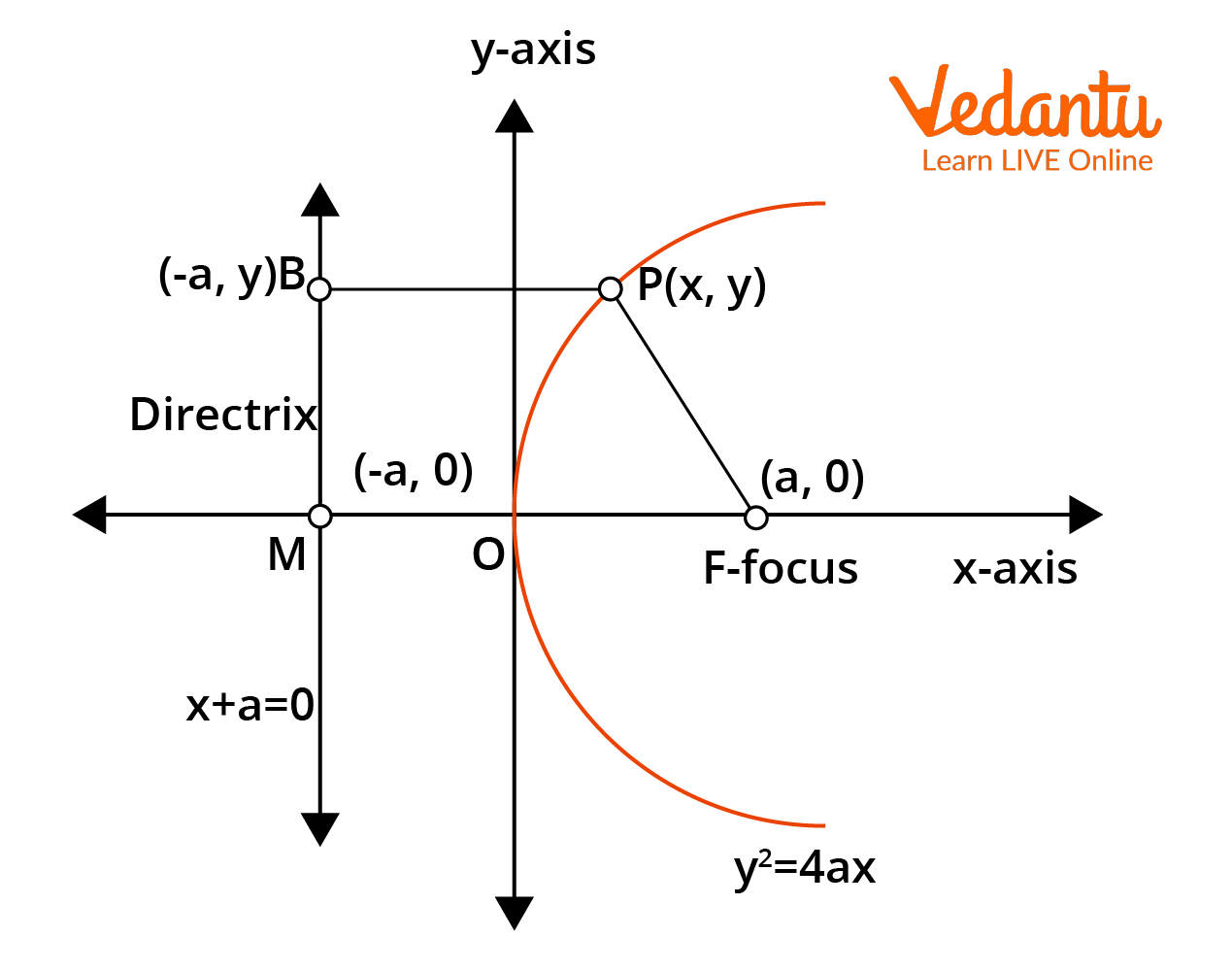Derivation of the Parabola Equation

The focus's coordinates are F(a,0). We can calculate its distance from P using the coordinate distance formula (x, y)

\begin{align} &P F=\sqrt{(x-a)^{2}+(y-0)^{2}} \\ &P F=\sqrt{(x-a)^{2}+(y)^{2}} \end{align}

The directrix's equation is x + a = 0, and we calculate PB using the perpendicular distance formula.

\begin{align} &P B=\dfrac{x+a}{\sqrt{1^{2}+0^{2}}} \\ &P B=x+a \\ &P B^{2}=\sqrt{(x+a)^{2}} \end{align}

We must use PF = PB to derive the parabola equation:

$\sqrt{(x-a)^{2}+y^{2}}=\sqrt{(x+a)^{2}}$

By squaring both sides of the equation,

\begin{align} &(x-a)^{2}+y^{2}=(x+a)^{2} \\ &x^{2}+a^{2}-2 a x+y^{2}=x^{2}+a^{2}+2 a x \\ &y^{2}=2 a x+2 a x \\ &y^{2}=4 a x \end{align}

We've now correctly deduced the typical parabola equation.

Now this was the equation of a parabola with its vertex at the origin. Suppose we have a parabola with its vertex at (h,k) then its equation is given as

$(y-k)^2=4a(x-h)$

We will see different types of parabolas in the next section. The equation of a parabola that we derived was opening towards the positive x-axis. There can be other parabolas opening towards the negative x-axis and the positive or negative y-axis.

## Parabola Equation in Standard Form

Cartesian coordinates are a pair of numerical coordinates that represent points on a plane (x, y)

1. ### If P is Greater than Zero and P is on the Positive Y-Axis:

$x^{2}=4 p y$

Here, coordinates of F = (0, p) lie on the y-axis and p is the distance from vertex to focus on the y-axis.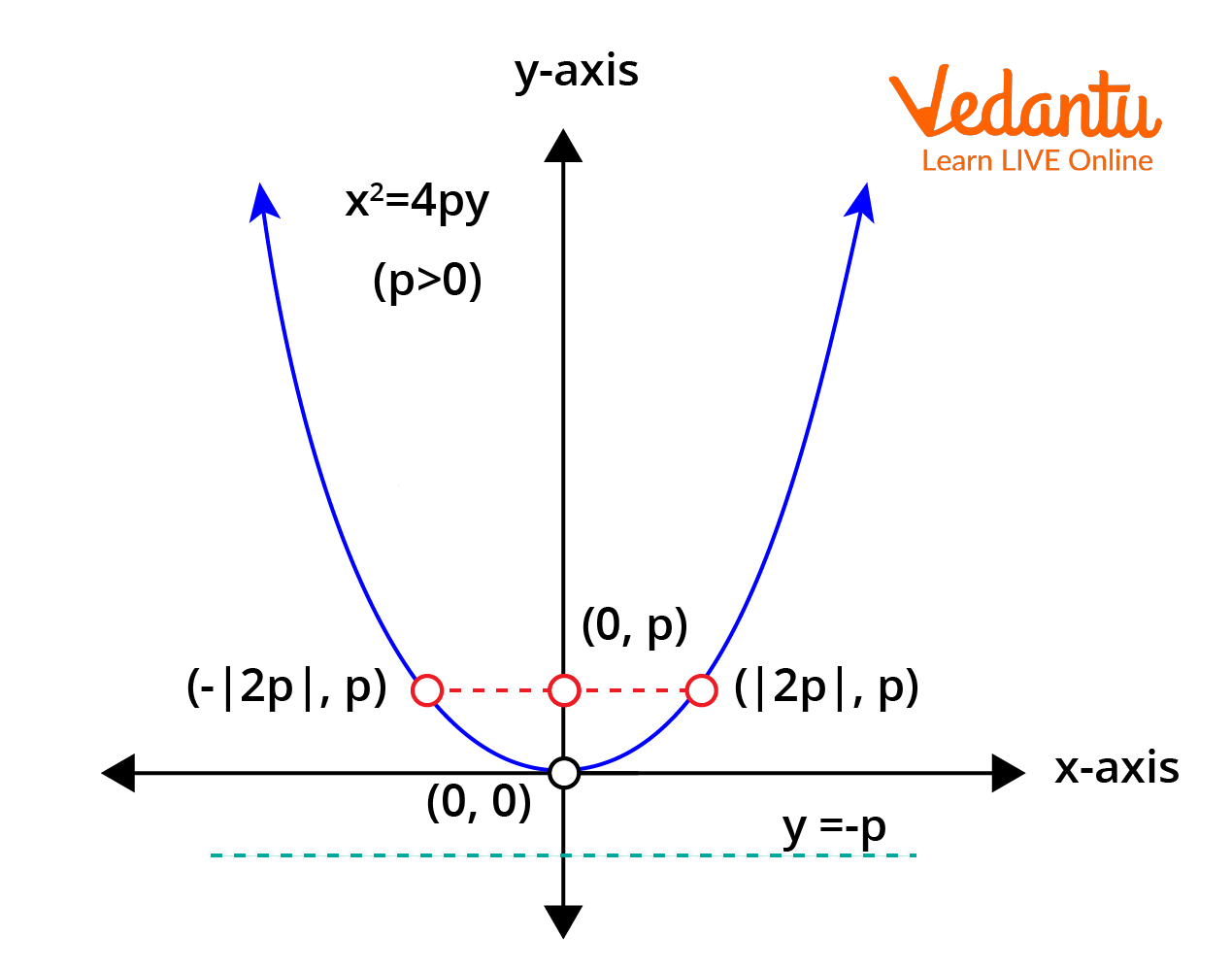Parabola x2 = 4 py with Coordinates of F = (0,P)

### (b) If P is Less than Zero and P is on the Negative X-Axis:

$y^{2}=-4 p x$

Here, coordinates of F = (-p, 0) lie on the y-axis and p is the distance from vertex to focus on the x-axis.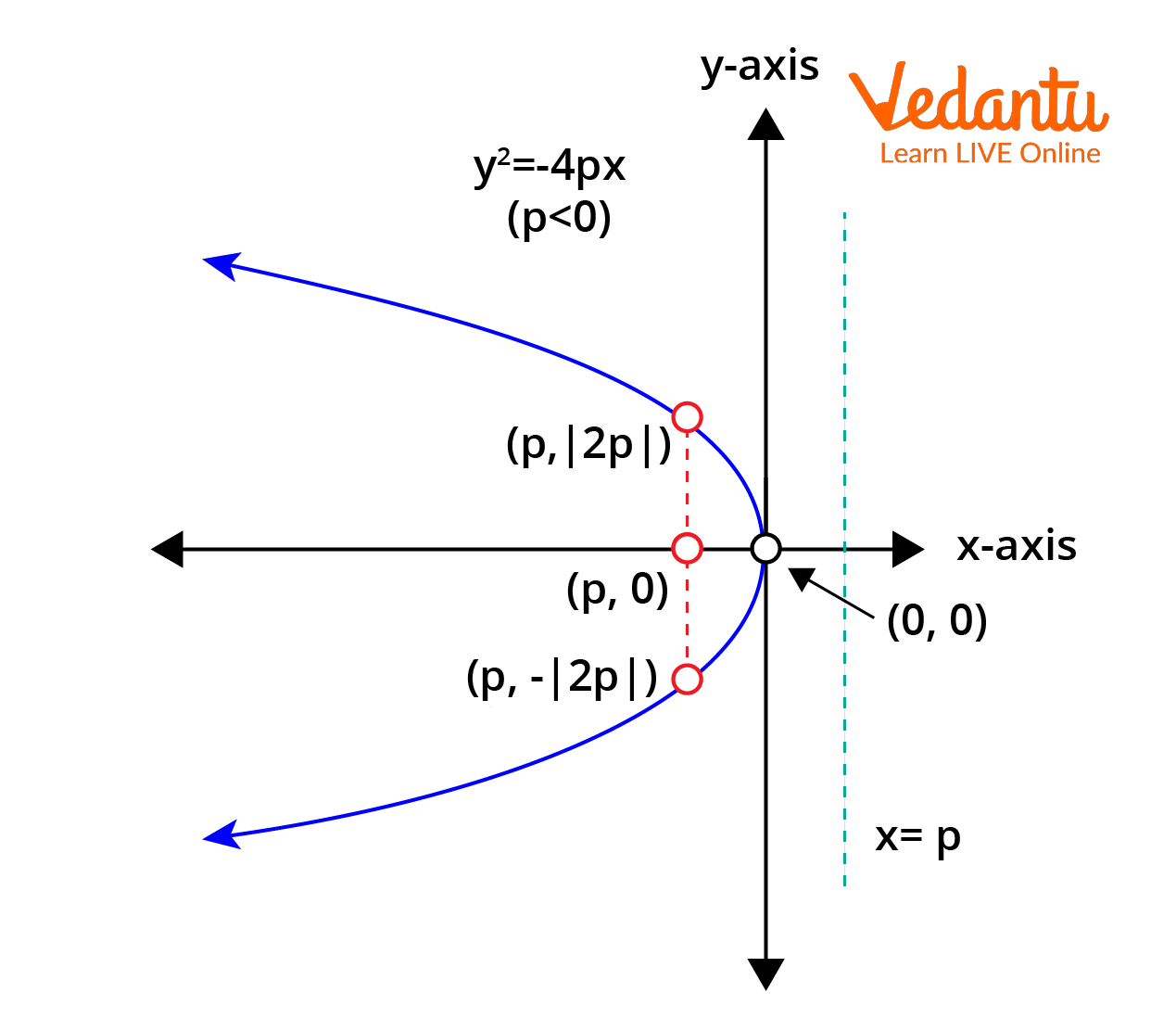Parabola with coordinates of F = (-P,0)

### (c) If P is Less than Zero and P is on the Negative Y-Axis:

$x^{2}=-4 p y$

Here, coordinates of F = (0, -p) lie on the y-axis and p is the distance from vertex to focus on the y-axis.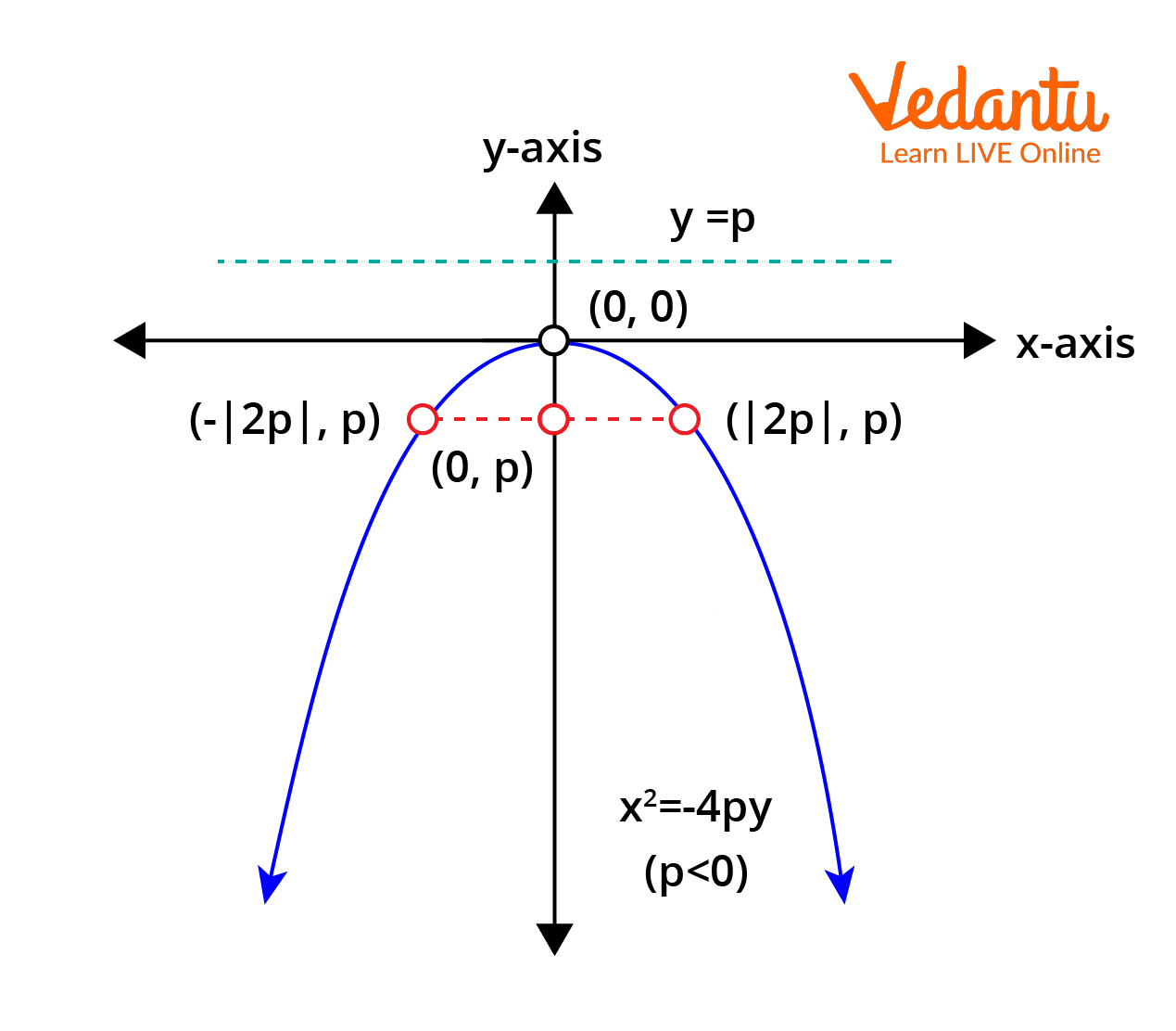Parabola with coordinates of F = (0,-P)

(d) If P is Greater than Zero and P is on the Positive X-Axis:

$y^{2}=4 p x$

Here, coordinates of F = (p, 0) lie on the y-axis and p is the distance from vertex to focus on the x-axis.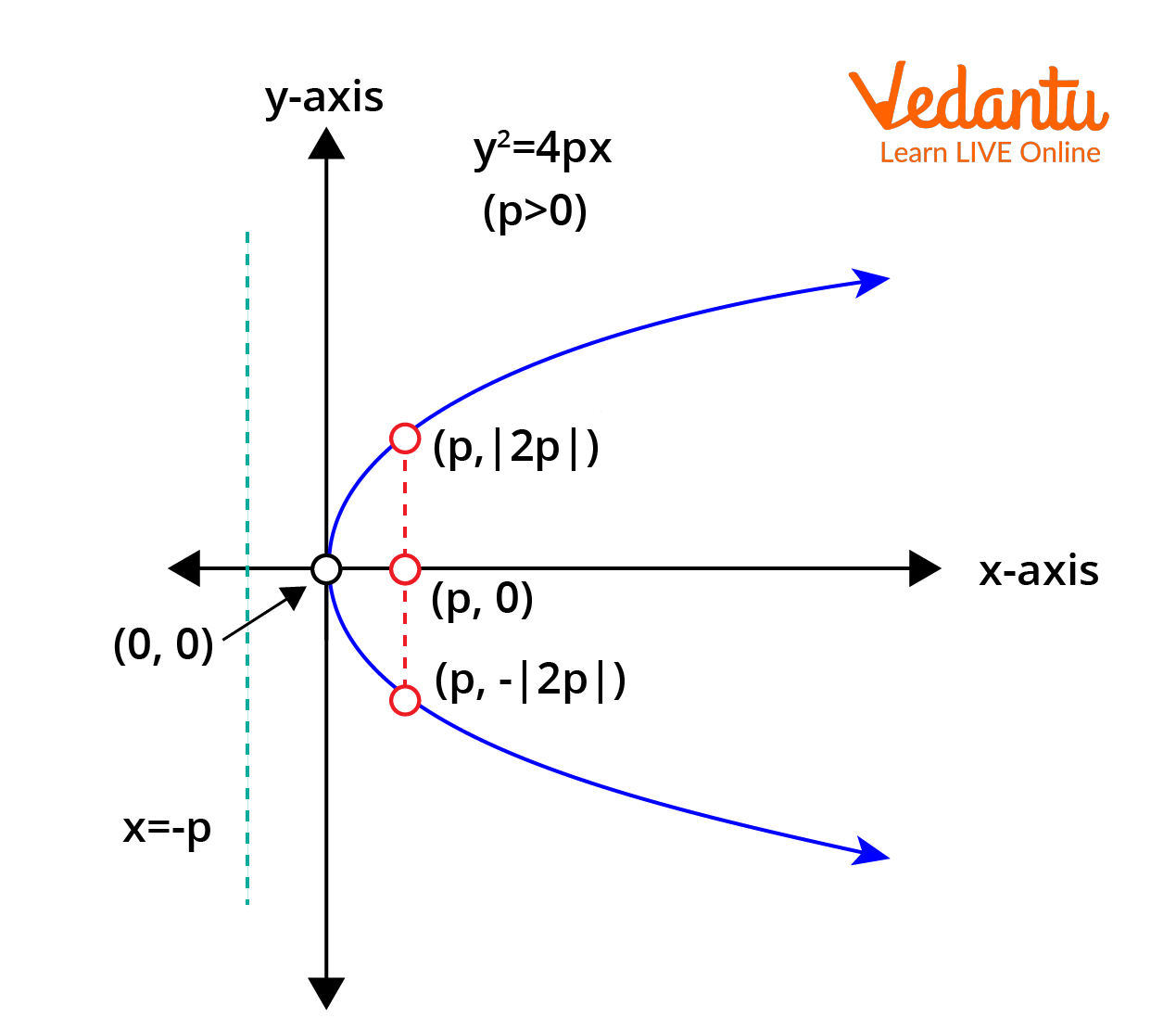Parabola with coordinates of F = (P,0)

The observations from the above section and the diagrams of the parabola have been summarised and listed below.

• A parabola has axis-symmetric geometry. The axis of symmetry runs along the x-axis if the equation contains the term with y2, and along the y-axis if the term with x2 is present in the equation.

• The parabola opens to the right if the coefficient of x is positive and to the left if the coefficient of x is negative when the axis of symmetry is along the x-axis.

• The parabola widens upwards if the coefficient of y is positive and downwards if the coefficient of y is negative when the axis of symmetry is along the y-axis.

## Parametric Equation of a Parabola

If we have a parabola with equation

y2 = 4ax , then the parametric equation of this parabola are

\begin{align} &x=a t^{2} \\ &y=2 a t \end{align}

Here t is the parameter of this parabola.

Any point on the parabola can be represented by the parametric equations as (at2, 2at)  and these points are called “t” points.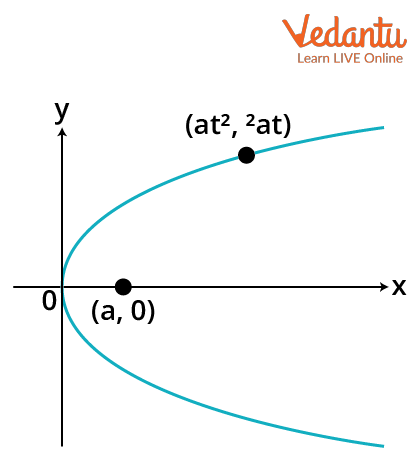Diagram showing the t point of a parabola

From the above image, we can see the diagram of a parabola with its t point and the focus represented by (a,0).

## Equation of a Chord Joining any Two Points of a Parabola

Suppose we have two points represented by the parameters t1 and t2 on a parabola. These points are given as (at12, 2at1)  and (at22, 2at2), then the equation of a chord joining these two points is given as,

\begin{align} &\left(y-2 a t_{1}\right)=\dfrac{2 a t_{2}-2 a t_{1}}{a t_{2}{ }^{2}-a t_{1}{ }^{2}}\left(x-a t_{1}{ }^{2}\right) \\ &y-2 a t_{1}=\dfrac{2 a\left(t_{2}-t_{1}\right)}{a\left(t_{1}+t_{2}\right)\left(t_{2}-t_{1}\right)}\left(x-a t_{1}{ }^{2}\right) \\ &y-2 a t_{1}=\dfrac{2}{t_{1}+t_{2}}\left(x-a t_{1}^{2}\right) \\ &\left(t_{1}+t_{2}\right) y=2 x+2 a t_{1} t_{2} \end{align}

A focal chord is a chord that passes through the focus of the parabola. For a focal chord,

t1t2=-1

Suppose now that one end of the focal chord is at (at2,2at) then the other end will be at $\left(\dfrac{a}{t^2},-2at\right)$

### Position of a Point with Respect to a Parabola

Suppose we have a point (x1,y1). Now to know if the point lies inside, outside, or on the parabola, we have to check whether, (y12-4ax1) is greater than, less than, or equal to zero.

• If y12-4ax1>0, then the point lies outside the region of the parabola.

• If y12-4ax1<0, then the point lies inside the region of the parabola.

Similarly if y12-4ax1=0, then the point lies on the parabola.

## Tangent to the Parabola

If a line represented by equation y=mx+c, touches a parabola y2=4ax then for the line to be a tangent to the parabola,

$c=\dfrac{a}{m}$

Similarly, if we have a parabola x2=4ay, then the line y=mx+c is a tangent to it if

$c=-am^2$

Now if the line is not given in the standard form then to check if the line is a tangent to a parabola, first, we need to eliminate one variable either x or y between the equations of the straight line and parabola and then apply the condition b2 = 4ac for the quadratic equation ax2+bx+c which is obtained.

The equation of a tangent to the parabola y2=4ax at a point (x1,y1) is given as

$yy_1=2a(x+x_1)$.

This equation is known as the point form equation of the tangent.

Similarly, the parametric form of a tangent to the parabola at a  point (at2,2at) is given as,

$ty=x+at^2$

The slope form of a tangent to a parabola y2=4ax is given as

$y=mx+\dfrac{a}{m}$

Here, m is the slope of the tangent.

## Point of Intersection of Two Tangents

Suppose we have two tangents to a parabola y2=4ax at points t1 and t2The equation of the tangent at t1 will be

$yt_1=x+at_1^2$ ….(1)

Similarly the equation of the tangent at point t2 will be

$yt_2=x+at_2^2$.....(2)

Now, if we subtract equations (1) and (2), we get $y(t_1-t_2)=a(t_1^2-t_2^2)$

\begin{align} &y\left(t_{1}-t_{2}\right)=a\left(t_{1}^{2}-t_{2}^{2}\right) \\ &y=\dfrac{a\left(t_{1}+t_{2}\right)\left(t_{1}-t_{2}\right)}{\left(t_{1}-t_{2}\right)} \\ &y=a\left(t_{1}+t_{2}\right) \end{align}

Putting this value of y in equation (1), we get

\begin{align} &a\left(t_{1}+t_{2}\right) t_{1}=x+a t_{1}^{2} \\ &a t_{1}^{2}+a t_{1} t_{2}=x+a t_{1}^{2} \\ &x=a t_{1} t_{2} \end{align}

So the point of intersection of the tangents has coordinates

$x=at_1t_2$

$y=a(t_1+t_2)$

So the point of intersection is

$(a_1t_2, a(t_1+t_2))$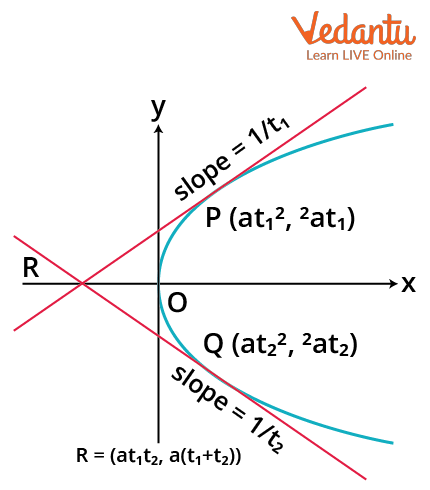Point of intersection of 2 tangents

## Normal to the Parabola

The tangent of a parabola is perpendicular to the parabola's normal.

Suppose we have a line y=mx+c and a parabola y2=4ax. This line is normal to the parabola when

$c=-2am-am^3$

Similarly, if the parabola is x2=4ay then the line y=mx+c is a normal to the parabola when

$c=2a+\dfrac{a}{m_2}$

## Equation of Normal

The equation of a normal at point (x1,y1) to the parabola y2=4ax is given as

$y-y_1=-\dfrac{y_1}{2a}(x-x_1)$

This is the point form of the normal.

The parametric form of the equation of a normal at point (at2,2at1) is

$y+tx=2at+at^3$

Similarly the slope point form of the normal in terms of slope m is given as

$y=mx-2am-am^3$

## Point of Intersection of Two Normals

Suppose we have two normals at points P(at12,2at1)  and Q(at22,2at2) on the parabola y2=4ax, then the point of intersection of the two normals represented by point R.

We know that normals and tangents are perpendicular to each other. As above mentioned that the slope of tangents is $\dfrac{1}{t_1}$ and $\dfrac{1}{t_2}$ so the slope of normals will be $-t_1$ and $-t_2$.

So writing equations of both normals;

$y-2a{t_1}=-{t_1}(x-a{t_1}^2)$ -----(1)

$y-2a{t_2}=-{t_2}(x-a{t_2}^2)$ -----(2)

Now we will solve equations (1) and (2) for finding the intersection point.

$2a{t_2}-{t_2}(x-a{t_2}^2)=2a{t_1}-{t_1}(x-a{t_1}^2)$

$x({t_2}-{t_1})=2a({t_2}-{t_1})+a({t_2}^3-{t_1}^3)$

$x=2a+a({t_2}^2+{t_1}^2+{t_1}{t_2})$

And substituting value of x in equation (1),

$y-2a{t_1}=-{t_1}(2a+a({t_2}^2+{t_1}^2+{t_1}{t_2})-a{t_1}^2)$

$y=2a{t_1}-{t_1}(2a+a{t_2}^2+a{t_1}{t_2})$

$y=-{t_1}(a{t_2}^2+a{t_1}{t_2})$

$y=-at_1t_2(t_1+t_2)$

The point of intersection of the normals is thus, $R(2a+a({t_2}^2+{t_1}^2+{t_1}{t_2}), -at_1t_2(t_1+t_2))$.

## Conclusion

The parabolic graph is a symmetrical figure which is a 2D curve that represents the graph of a quadratic equation. Any point on the parabola maintains a fixed distance from the focus (point) and the directrix (straight line). The focal chord is any straight line passing through the focus of the parabola. The vertex of the parabola is the point of intersection between the parabolic graph and the line of symmetry. The slope of the normal of a parabola is the inverse of the slope of the tangent of the parabola. The general equation of a parabola is given by $y^{2}=4 a x$, where the vertex is at the origin (0, 0) and the axis is the y-axis.

Last updated date: 22nd Sep 2023
Total views: 128.4k
Views today: 3.28k

## FAQs on Parabola General Equations - General Equations for JEE

1. Where can parabolas be seen in real life?

There are a lot of real-life parabola examples that one encounters in everyday life. If you observe carefully, most bridges and flyovers are parabolic in nature. If you throw a ball upwards, the path taken by the ball before touching the ground is also a parabola. The roller coasters in the amusement parks are also classic real-life examples of the parabolic curve. Parabola has utilities in other fields as well. In economics, most of the profits, losses and forecasts are denoted by the parabolic graph. The height of the parabola represents the profits. The bigger the winnings, the higher the parabola.

2. What significance does the current topic have in terms of board exams? How much weight does it have in the JEE exam?

In the board exam, the topic parabola has intermediate levels. Since the board test is subjective in nature, the theoretical and numerical sections of this text are critical from an exam standpoint. Some numerical information is also available from the score advantage section. The weightage of the parabola is moderate because it only comprises a small amount of mathematics. The parabola is a simple idea that is used in this topic. Every year 2 to 3 questions from the parabola topic are asked in the JEE Main.